Create a new printable

Black and white graphics
Color graphics

Select Currency
 US Dollar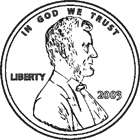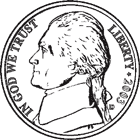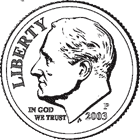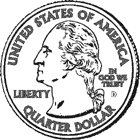Canadian Dollar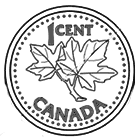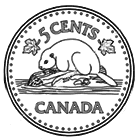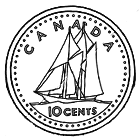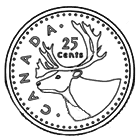Euro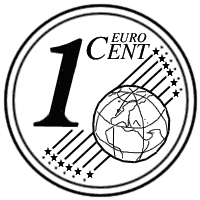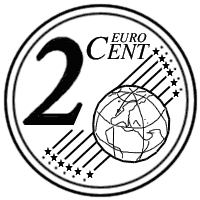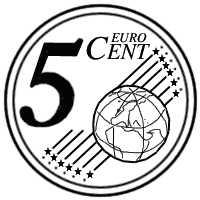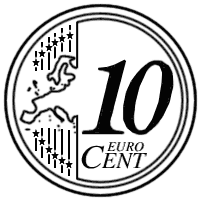Math Worksheets

Sample - Click above to make a new math worksheet (PDF).
 Name _____________________________Date ___________________
Pennies, Nickels, and Dimes
Fill in the missing value.

1.
 1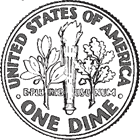+ 9+ 8= _____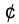1 dime  + 9 nickels  + 8 pennies  = _____2 * This is a pre-made sheet.Use the link at the top of the page for a printable page.
3.
 _____+ 5+ 1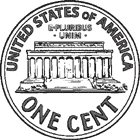= 96_____ nickels  + 5 dimes  + 1 penny  = 964.
 3+ _____+ 4= 583 dimes  + _____ pennies  + 4 nickels  = 585.
 _____+ 4+ 8= 48_____ dimes  + 4 nickels  + 8 pennies  = 48Sample
This is only a sample worksheet.

Create a new printable
 US DollarCanadian DollarEuro